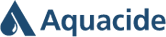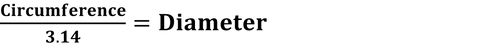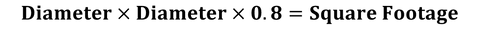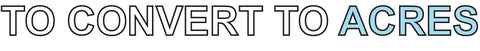Circular Calculation - Aquacide

#Call us today! 1-800-328-9350

### From the Blog1.Pace off the Circumference of the body of water (in feet.) The circumference of a circle is the linear distance of a circle's edge. It is the same as perimeter.2.Divide the Circumference by Pi (3.14) to determine the Diameter. The Diameter is a straight line passing from side to side through the center of a circle.3. Square the Diameter (in feet.) and multiply by 0.84. Divide the Square Footage by 43,560 (the square feet in an acre) to determine the Acreage.